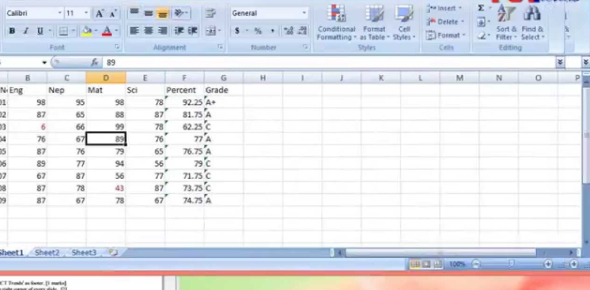# The Microsoft Excel Exam: MCQ Quiz

20 Questions | Total Attempts: 665SettingsCreate your own QuizCan you pass this Microsoft Excel MCQ Test? MS. Excel is one of the best tools for someone who wants to use and organize different complex data sets. The use of formulas makes it easy to analyze data very fast and is also one of the challenging parts for many when it comes to many users. Do you think you have the perfect understanding of how to make the most use of this tool? Test yourself!

• 1.
V\:* {behavior:url(#default#VML);} o\:* {behavior:url(#default#VML);} w\:* {behavior:url(#default#VML);} .shape {behavior:url(#default#VML);} A fast way to add up this column of numbers is to click in the cell below the numbers and then:
• A.

Click Subtotals on the Data menu.

• B.

View the sum in the formula bar.

• C.

Click the AutoSum button on the Standard toolbar, then press ENTER.

• 2.
If you want to paste a formula result — but not the underlying formula — to another cell. You would copy the cell with the formula, then place the insertion point in the cell you want to copy to. What next?
• A.

Click the Paste button on the Standard toolbar.

• B.

Click the arrow on the Paste button on the Standard toolbar, then click Formulas.

• C.

Click the arrow on the Paste button on the Standard toolbar, then click Values.

• 3.
How do you change the column width to fit the contents?
• A.

Single-click the boundary to the left of the column heading.

• B.

Double-click the boundary to the right of the column heading.

• C.

Press ALT and single-click anywhere in the column.

• 4.
There are three worksheets with every new workbook. You can change that automatic number if you want to.
• A.

True

• B.

False

• 5.
###### means:
• A.

You've entered a number wrong.

• B.

You've misspelled something.

• C.

The cell is not wide enough.

• 6.
To add a new row, click a cell in the row immediately above where you want the new row.
• A.

True

• B.

False

• 7.
If you want to perform an operation that adds the contents of cells which formulas would you use?
• A.

=Sum(a1:B1)

• B.

=Average(A1:B1)

• C.

=A1+B1

• 8.
To copy an entire worksheet and all its data, you should click the worksheet tab of the sheet that you want to copy, hold down SHIFT, and then drag the selected sheet along the row of sheet tabs.
• A.

True

• B.

False

• 9.
A user wishes to remove a spreadsheet from a workbook. Which is the correct sequence of events that will do this?
• A.

Go to FILE - SAVE AS - SAVE AS TYPE - Excel 4.0 Work Sheet

• B.

Right click on the spreadsheet tab and select DELETE

• C.

Right click on the spreadsheet and select INSERT - ENTIRE COLUMN

• 10.
Which formula can add all the numeric values in a range of cells, ignoring those which are not numeric, and place the result in a different cell?
• A.

Count

• B.

Average

• C.

Sum

• 11.
Is it possible to insert an image from a file into an Excel spreadsheet?
• A.

Yes

• B.

No

• 12.
Can an Excel spreadsheet be used as the "data source" for a Word Mail Merge?
• A.

Yes

• B.

No

• 13.
On an Excel sheet, the active cell is indicated by ____
• A.

A dark wide border

• B.

A dotted border

• C.

• D.

None of the above

• 14.
A Formula and a function are the same things.
• A.

True

• B.

False

• 15.
In order to multiply items in Excel you would use:
• A.

^

• B.

@

• C.

*

• D.

#

• 16.
The formula = ((A2+B5)*5% is valid.
• A.

True

• B.

False

• 17.
If cells: A1=90 A2=85 A3=80 A4=75 A5=75 What will be your formula if you are going to get the average?
• 18.
If cells: A1=90 A2=85 A3=80 A4=75 A5=75 What will be your formula if you are going to get the total?
• 19.
If you wanted to insert a chart into an excel spreadsheet what 3 steps would you follow?
• 20.
What steps would you take to insert a header into an excel worksheet?
Related TopicsBack to top
×

Wait!
Here's an interesting quiz for you.